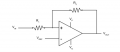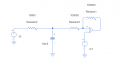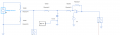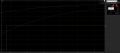# Understanding the math behind a simple circuit

#### printedcircuits

Joined Sep 18, 2023
8
Hello
I am a student and my English is limited so i apologize. This is my first post. I hope its ok. I wish to understand the interaction between a comparator and an RC circuit from a mathematical point.
I think we all know what a time constant is and the governing equations. The thing that troubles me so, is in the case where you have a non inverting comparator with positve feedback with an RC timer on the input.

What i would like to understand more in depth is how to calculate at how many seconds it will take for the RC timer to hit the reference voltage threshold. Normally, i would of just gone to the normal equations we all of

Vc(t) = V(1-e^(-t/rc) and solved for the t = time with the voltages i want.

However, when you add the positive feedback of a circuit like this, this equation no longer holds true. Because of the additional resistors.
Im trying to develop an equation like this that does work, but works correctly when you have positive feedback resistors in play.

The below picture is borrowed from this forum. This is the configuration. However, it is not show with a pullup resistor which also still must be added.If i take this circuit and put an RC timer in front of it going into the input, the time it would take to go over the reference voltage is what i want to understand. The standard equation no longer holds true because of the additional positive feedback network.

Nor do the hysteresis equations hold true anymore.
Here is an example of the circuit i speak of.Could someone point me to where i may find an example of the math to understand how to modify this equation so it works in these situation?
Vc(t) = V(1-e^(-t/rc)

#### MrChips

Joined Oct 2, 2009
29,809
Welcome to AAC!

Ignore resistors R1 and R3 and calculate using the normal RC equation.
The purpose of the comparator with positive feedback is to establish hystersis. You can calculate the rising and falling switching thresholds by simple DC analysis.

Since R3 is ten times larger than R2 its effect would be minimal.

#### printedcircuits

Joined Sep 18, 2023
8
Welcome to AAC!

Ignore resistors R1 and R3 and calculate using the normal RC equation.
The purpose of the comparator with positive feedback is to establish hystersis. You can calculate the rising and falling switching thresholds by simple DC analysis.

Since R3 is ten times larger than R2 its effect would be minimal.
Hi, thank you for your kind reply. I intentionally made R3 10X bigger so it wont effect it.
I realized i made a mistake in my original question.

With the basic circuit i posted above, your suggestion works.
However, it does not work if i add a voltage divider in front of the RC circuit.
please excuse the little voltage blocks. That is how my simulator does voltage measurements.
When i add the resistor divider the equation no longer holds true. Can you tell me why that is or how to include that into my math?#### WBahn

Joined Mar 31, 2012
29,489
The problem with the divider is that it's effective resistance, as seen by R2, is not negligible.

I assume you are treating the divider as producing a constant 10 V signal into the RC portion of the circuit. Unfortunately, that 10 V is only going to be the case if whatever current is flowing in R4 also flows in R5 -- which means that no current flows in R2.

If R4 and R5 are sufficiently small compared to R2, then the current that leaves the voltage divider output is small compared to the currents in R4 and R5 and the assumption that the output is a constant 10 V is much better. To achieve this, you need R4 and R5 to be about 100x smaller than R2 (although just 10x smaller will yield a significant improvement).

If you are dealing with RC circuits, you should have already been exposed to Thevenin equivalent circuits. The 20 V source and the R4, R5 voltage divider are equivalent to an ideal 10 V source with a series output resistance of 5000 Ω. So redo your calculations with that in mind and see how they work out.

#### printedcircuits

Joined Sep 18, 2023
8
The problem with the divider is that it's effective resistance, as seen by R2, is not negligible.

I assume you are treating the divider as producing a constant 10 V signal into the RC portion of the circuit. Unfortunately, that 10 V is only going to be the case if whatever current is flowing in R4 also flows in R5 -- which means that no current flows in R2.

If R4 and R5 are sufficiently small compared to R2, then the current that leaves the voltage divider output is small compared to the currents in R4 and R5 and the assumption that the output is a constant 10 V is much better. To achieve this, you need R4 and R5 to be about 100x smaller than R2 (although just 10x smaller will yield a significant improvement).

If you are dealing with RC circuits, you should have already been exposed to Thevenin equivalent circuits. The 20 V source and the R4, R5 voltage divider are equivalent to an ideal 10 V source with a series output resistance of 5000 Ω. So redo your calculations with that in mind and see how they work out.
Thanks
I got a closer answer but I'm still off by about 20mS which is frustrating. Is there a more accurate way?

#### WBahn

Joined Mar 31, 2012
29,489
Thanks
I got a closer answer but I'm still off by about 20mS which is frustrating. Is there a more accurate way?
There's no way I can tell why your calculations are off by 20 ms since you don't show any calculations for anyone to review.

What is the output swing of your comparator? That is key to determining what voltage the capacitor has to get to in order to reach the comparator's trip point. Without that information, there's no way to tell.

#### printedcircuits

Joined Sep 18, 2023
8
There's no way I can tell why your calculations are off by 20 ms since you don't show any calculations for anyone to review.

What is the output swing of your comparator? That is key to determining what voltage the capacitor has to get to in order to reach the comparator's trip point. Without that information, there's no way to tell.
Hi

I am not considering the comparator at this time. All im doing is using this equation
Vc(t) = V(1-e^(-t/rc)

I am saying V = Vth as you suggested.
r = Rth+10k
and Vc im just picking at random.
The whole thing is solved for t to tell me how many seconds it should take to get to a certain Vc voltage.

Im looking at it from the math and then wondering my the simulation is does not match.

so for example,

3.3V = 10V*(1-e^(-t/(15k*10uF)
where the 15k is the sum if rth and the resistor in front of the cap. the cap being 10uf.

#### WBahn

Joined Mar 31, 2012
29,489
So how do you know that your simulation doesn't match? The schematic you show has a volt meter at the output of the voltage divider and at the output of the comparator. But you don't have anything measuring the voltage across the capacitor, which is what your equation is for.

The comparator won't trip when the capacitor voltage is 3.3 V due to the hysteresis.

How does your time compare to when the capacitor voltage reaches 3.3 V?

#### dl324

Joined Mar 30, 2015
16,113
Your equations both have mistakes. It's be easier to catch them if you use Tex.
I am not considering the comparator at this time. All im doing is using this equationΩ
Vc(t) = V(1-e^(-t/rc)
You're missing one right parenthesis. This is what you meant:
$$\large V_C = V(1-e^\frac{-t}{RC})$$
3.3V = 10V*(1-e^(-t/(15k*10uF)
where the 15k is the sum if rth and the resistor in front of the cap. the cap being 10uf.
This equation is missing 2 right parenthesis. This is what you meant to write:
$$\large 3.3V = 10V*(1-e^\frac{-t}{15kΩ*10uF})$$

#### WBahn

Joined Mar 31, 2012
29,489
The first equation is missing a critical set of parens since -t/rc is NOT the same as -t/(rc). But I chose to set that aside for the moment.

#### printedcircuits

Joined Sep 18, 2023
8
Sorry about the text formatting. I was not aware that was a thing.
I understand about the hysteresis. However, The cap voltage rising does not equal the equation by a factor of 2 at least.

#### WBahn

Joined Mar 31, 2012
29,489
Sorry about the text formatting. I was not aware that was a thing.
I understand about the hysteresis. However, The cap voltage rising does not equal the equation by a factor of 2 at least.
I'm still operating in a vacuum here, because I don't know what you are measuring, what you are measuring it relative to, what you are expecting to see, and what you are actually seeing.

In an earlier post, you said you were still of by 20 ms. WHAT is off by 20 ms? The time from what event to what event? Off in which direction? Is the simulated result 20 ms longer or shorter than expected? What was the time that was expected?

Now you say that it doesn't equal the equation by a factor of 2. A factor of two with regards to what? Voltage? Time? Is the simulation a factor of two too large? Or too small?

Please show the actual simulation results. Annotate them with what you believe is not in agreement with your calculations, and note what your calculations say the results should be.

Otherwise, it's like calling up the doctor and asking him to diagnose your condition and all you will tell him is that you aren't sleeping the right amount of time.

#### dl324

Joined Mar 30, 2015
16,113
Sorry about the text formatting. I was not aware that was a thing.
Equations in simple text are more difficult to read. Better formatting would have let you see your mistakes.

Upper/lower case is also a thing. Farads are abbreviated F, not f. You used both...

The cap voltage rising does not equal the equation by a factor of 2 at least.
The lack of "stiffness" in your voltage divider caught my eye. But your schematic has too much whitespace and color for me to think seriously about trying to analyze it.

#### WBahn

Joined Mar 31, 2012
29,489
The lack of "stiffness" in your voltage divider caught my eye. But your schematic has too much whitespace and color for me to think seriously about trying to analyze it.
The divider compliance has been addressed -- he is now incorporating the 5 kΩ output resistance in his calculations.

#### printedcircuits

Joined Sep 18, 2023
8
The divider compliance has been addressed -- he is now incorporating the 5 kΩ output resistance in his calculations.
I will work on adding another picture

When i say im off what i mean is the following. If i use the equation you all corrected for me above and i pick any voltage and solve for the time t that it should be at that voltage based on the Vth voltage of 10v the simulation shows to be off a good bit and that is what im not understanding

So say for example like i said above, i picked 3.3 just because that is what the reference is.
3.3V=10V∗(1−e−t15kΩ∗10uF)
solving for t i get 60mS
So if i look at the plot and cursor over 3.3V at the cap, i expect that to happen at 60mS
When i look at the simulation it shows 150mS
Thats what i meant by off about by 2Last edited:

#### printedcircuits

Joined Sep 18, 2023
8
I will work on adding another picture

When i say im off what i mean is the following. If i use the equation you all corrected for me above and i pick any voltage and solve for the time t that it should be at that voltage based on the Vth voltage of 10v the simulation shows to be off a good bit and that is what im not understanding

So say for example like i said above, i picked 3.3 just because that is what the reference is.
3.3V=10V∗(1−e−t15kΩ∗10uF)
solving for t i get 60mS
So if i look at the plot and cursor over 3.3V at the cap, i expect that to happen at 60mS
When i look at the simulation it shows 150mS
Thats what i meant by off about by 2

View attachment 303064
Also, as a side note. When i take the comparator out, it works as expected. There is some effect from the positive feedback i dont understand.

#### dl324

Joined Mar 30, 2015
16,113
3.3V=10V∗(1−e−t15kΩ∗10uF)
You forgot exponentiation and division operators this time.

#### printedcircuits

Joined Sep 18, 2023
8
You forgot exponentiation and division operators this time.
Thats because i copy and pasted your text and it didnt work
Its fine, i have a solution to my problem
Very many thanks to all

#### dl324

Joined Mar 30, 2015
16,113
When i take the comparator out, it works as expected.
What device are you using for the comparator?
Thats because i copy and pasted your text and it didnt work
And because of that, you couldn't enter this?
3.3V=10V∗(1−e^(−t/(15kΩ∗10uF)))

#### WBahn

Joined Mar 31, 2012
29,489
Also, as a side note. When i take the comparator out, it works as expected. There is some effect from the positive feedback i dont understand.
Plot the current in the 100 kΩ and see if it is more than expected.

What simulator are you using?